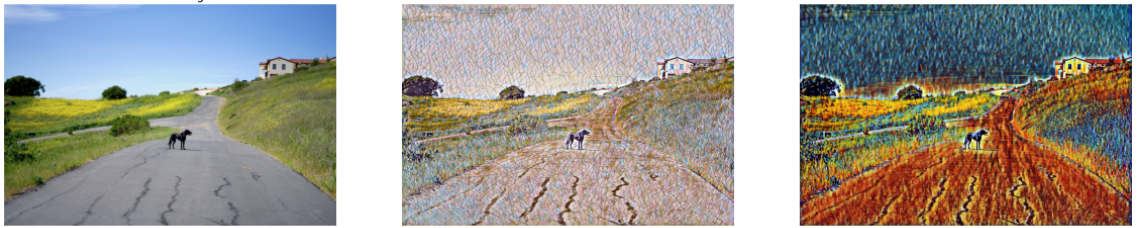# Style Transfer on ONNX Models with OpenVINO™¶

This tutorial is also available as a Jupyter notebook that can be cloned directly from GitHub. See the installation guide for instructions to run this tutorial locally on Windows, Linux or macOS. To run without installing anything, click the launch binder button.Neural Style Transfer network output

This notebook demonstrates Fast Neural Style Transfer on ONNX models with OpenVINO. Style Transfer models mix the content of an image with the style of another image.

This notebook uses five pre-trained models, for the following styles: Mosaic, Rain Princess, Candy, Udnie and Pointilism. The models are from the ONNX Model Repository and are based on the research paper Perceptual Losses for Real-Time Style Transfer and Super-Resolution by Justin Johnson, Alexandre Alahi and Li Fei-Fei.

## Preparation¶

### Imports¶

import sys
from enum import Enum
from pathlib import Path

import cv2
import matplotlib.pyplot as plt
import numpy as np
from IPython.display import HTML, FileLink, clear_output, display
from openvino.runtime import Core, PartialShape
from yaspin import yaspin

sys.path.append("../utils")


The Style Enum lists the supported styles with url, title and model_path properties. Models for all supported styles will be downloaded to MODEL_DIR if they have not been downloaded before.

BASE_URL = "https://github.com/onnx/models/raw/main/vision/style_transfer/fast_neural_style/model"
MODEL_DIR = "model"

class Style(Enum):
MOSAIC = "mosaic"
RAIN_PRINCESS = "rain-princess"
CANDY = "candy"
UDNIE = "udnie"
POINTILISM = "pointilism"

def __init__(self, *args):
self.model_path = Path(f"{self.value}-9.onnx")
self.title = self.value.replace("-", " ").title()
self.url = f"{BASE_URL}/{self.model_path}"

for style in Style:
if not Path(f"{MODEL_DIR}/{style.model_path}").exists():

model/mosaic-9.onnx:   0%|          | 0.00/6.42M [00:00<?, ?B/s]

model/rain-princess-9.onnx:   0%|          | 0.00/6.42M [00:00<?, ?B/s]

model/candy-9.onnx:   0%|          | 0.00/6.42M [00:00<?, ?B/s]

model/udnie-9.onnx:   0%|          | 0.00/6.42M [00:00<?, ?B/s]

model/pointilism-9.onnx:   0%|          | 0.00/6.42M [00:00<?, ?B/s]


Load an image with OpenCV and convert it to RGB. The style transfer model will be resized to the image shape. This gives the most detailed results, but for larger images, inference will take longer and use more memory. The resize_to_max function optionally resizes the image to a maximum size.

IMAGE_FILE = "data/coco_square.jpg"

def resize_to_max(image: np.ndarray, max_side: int) -> np.ndarray:
"""
Resize image to an image where the largest side has a maximum length of max_side
while keeping aspect ratio. Example: if an original image has width and height of (1000, 500)
and max_side is 300, the resized image will have a width and height of (300, 150).

:param image: Array of image to resize
:param max_side: Maximum length of largest image side
:return: Resized image
"""
if max(image.shape) <= max_side:
new_image = image
else:
index = np.argmax(image.shape)
factor = max_side / image.shape[index]
height, width = image.shape[:2]
new_height, new_width = int(factor * height), int(factor * width)
new_image = cv2.resize(image, (new_width, new_height))
return new_image

# Uncomment the line below to resize large images to a max side length to improve inference speed.
# image = resize_to_max(image=image, max_side=1024)


## Do Inference and Show Results¶

For all five models: do inference, convert the result to an 8-bit image, show the results, and save the results to a disk.

# Set SAVE_RESULTS to False to disable saving the result images.
SAVE_RESULTS = True

# Find reasonable dimensions for a matplotlib plot.
wh_ratio = image.shape / image.shape
figwidth = 15
figheight = (figwidth * 0.75) // wh_ratio

# Create a matplotlib plot and show the source image.
fig, ax = plt.subplots(2, 3, figsize=(figwidth, figheight))
axs = ax.ravel()
axs.imshow(image)
axs.set_title("Source Image")
axs.axis("off")

# Create a Core instance, prepare an output folder.
ie = Core()
output_folder = Path("output")
output_folder.mkdir(exist_ok=True)

# Transpose the input image to network dimensions and extract the name and the shape of the image.
input_image = np.expand_dims(image.transpose(2, 0, 1), axis=0)
image_name = Path(IMAGE_FILE).stem
image_shape_str = f"{image.shape}x{image.shape}"

for i, style in enumerate(Style):
# Load the model and get model info.
input_key = model.input(0)

# Reshape the network to the image shape and load the network to a device.
model.reshape({input_key: PartialShape([1, 3, image.shape, image.shape])})
compiled_model = ie.compile_model(model=model, device_name="CPU")
output_key = compiled_model.output(0)
# Do inference.
with yaspin(text=f"Doing inference on {style.title} model") as sp:
result = compiled_model([input_image])[output_key]
sp.ok("✔")

# Convert the inference result to the image shape and apply postprocessing.
# Postprocessing is described in the model documentation:
# https://github.com/onnx/models/tree/master/vision/style_transfer/fast_neural_style
result = result.squeeze().transpose(1, 2, 0)
result = np.clip(result, 0, 255).astype(np.uint8)

# Show the result.
axs[i + 1].imshow(result)
axs[i + 1].set_title(style.title)
axs[i + 1].axis("off")

# (optional) Save the results to a disk.
if SAVE_RESULTS:
image_path = f"{image_name}_{style.model_path.stem}_{image_shape_str}.png"
output_path = output_folder / image_path
cv2.imwrite(str(output_path), cv2.cvtColor(result, cv2.COLOR_BGR2RGB))

del model
del compiled_model

clear_output(wait=True)
fig.tight_layout()
plt.show()

if SAVE_RESULTS:
output_path = output_folder / f"{image_name}_{image_shape_str}_style_transfer.jpg"Saved image files:Mosaic image: output/coco_square_mosaic-9_2048x1366.png